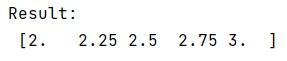# Is there a multi-dimensional version of arange/linspace in numpy?

Learn about the multi-dimensional version of arange/linspace in numpy?
Submitted by Pranit Sharma, on January 10, 2023

NumPy is an abbreviated form of Numerical Python. It is used for different types of scientific operations in python. Numpy is a vast library in python which is used for almost every kind of scientific or mathematical operation. It is itself an array which is a collection of various methods and functions for processing the arrays.

## Multi-dimensional version of arange/linspace in numpy

This method returns evenly spaced numbers over a specified interval. Also, it returns num evenly spaced samples, calculated over the interval [start, stop]. The endpoint of the interval can optionally be excluded.

It takes arguments like start and stop which are the starting value of the sequence and the end value of the sequence respectively. The end value unless endpoint is set to False. In that case, the sequence consists of all but the last of num+1 evenly spaced samples, so that stop is excluded. Note that the step size changes when endpoint is False.

Let us understand with the help of an example,

## Python code to demonstrate about the multi-dimensional version of arange/linspace in numpy

```# Import numpy
import numpy as np

# Using linspace method
res = np.linspace(2.0, 3.0, num=5)

# Display result
print("Result:\n",res,"\n")
```

Output: